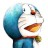# 杰拉斯的博客

## [ACM_NYOJ_8]一种排序杰拉斯 | 时间：2013-05-05, Sun | 22,428 views

### 一种排序

#### 描述

1.按照编号从小到大排序

2.对于编号相等的长方形，按照长方形的长排序；

3.如果编号和长都相同，按照长方形的宽排序；

4.如果编号、长、宽都相同，就只保留一个长方形用于排序,删除多余的长方形；最后排好序按照指定格式显示所有的长方形；

```1
8
1 1 1
1 1 1
1 1 2
1 2 1
1 2 2
2 1 1
2 1 2
2 2 1
```

```1 1 1
1 2 1
1 2 2
2 1 1
2 2 1
```

#### 来源

NYOJ

```#include<stdio.h>
#include<vector>
#include<algorithm>

using namespace std;

struct s{
int id, a, b;
s(int id, int a, int b):id(id),a(a),b(b){};
};

bool cmp(s s1, s s2){
if(s1.id != s2.id){
return s1.id < s2.id;
}
if(s1.a != s2.a){
return s1.a < s2.a;
}
return s1.b < s2.b;
}

int main(){
int t;
scanf("%d", &t);
while(t--){
int n, id, a, b;
vector<s> v;
scanf("%d", &n);
while(n--){
scanf("%d%d%d", &id, &a, &b);
if(a < b){
a = a ^ b;
b = b ^ a;
a = a ^ b;
}
v.push_back(s(id, a, b));
}
sort(v.begin(), v.end(), cmp);
id = 0; a = 0; b = 0;
for(vector<s>::iterator it = v.begin(); it != v.end(); ++it){
if(id != (*it).id || a != (*it).a || b!= (*it).b){
id = (*it).id;
a = (*it).a;
b = (*it).b;
printf("%d %d %d\n", id, a, b);
}
}
}
return 0;
}
```